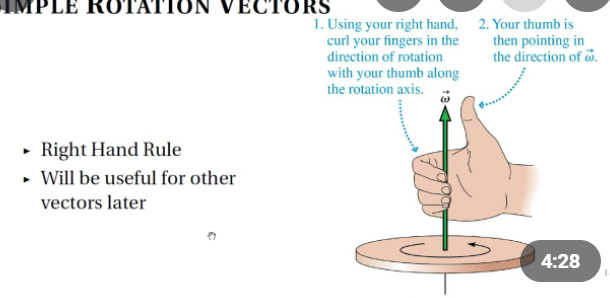# Angular velocity in direction

I want to roll a ball with angular velocity in the direction of the camera look vector. How would i do this, iv tried adding the look vector to the velocity but its not going in the right direction!

Angular velocity uses the Right Hand rule. The thumb is the direction of the angular velocity vector and the curl is the resulting direction of the rotation.Consequently, use the cameras right vector instead and multiply it by -1 and a constant speed value (to make it faster) to get a clockwise forward movement.

``````local speed = 100
thing.AngularVelocity = camera.CFrame.RightVector*-1*speed
``````
1 Like